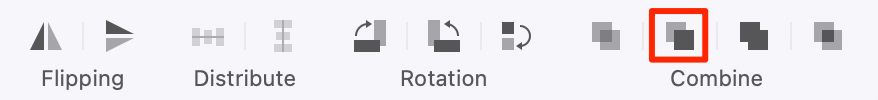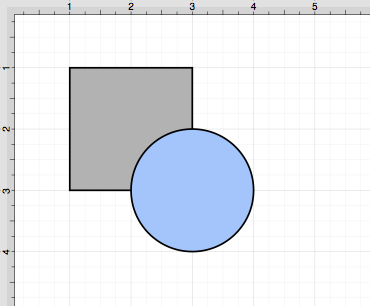# Subtract Shapes

The Subtract Shapes option removes the intersecting portions of the second selected shape from the first figure selected in the drawing canvas. This option remains disabled until two figures have been selected. Use one of the following methods to Subtract selected shapes:

1. Select the Modify Menu and choose Subtract Shapes.2. Use the Subtract Shapes button located on the main toolbar.Note: The selection of two non-intersecting shapes causes the second shape to be removed entirely.

The properties of the first selected shape will be applied to the combined shape. In the example below, the circle is selected first.The following image shows the resulting shape after using the Subtract Shapes option.